Archive for December 29th, 2008

Hyperion Essbase 9.3.1 – Alternative Approach to Currency Conversion in BSO Cubes – Using Calculation Scripts – Part 2

Posted by Venkatakrishnan J on December 29, 2008

In the last blog entry we saw how to go about designing the Essbase Outline to accomodate Rate loads into the same database. Today we shall look at how the currency conversion is accomplished. To begin with let us load some sample data into the Essbase Cube. The sample data is shown belowAs you see, the input data is in Local currency of each city/country. Let us create a simple rule which will load the above data into the Essbase Cube.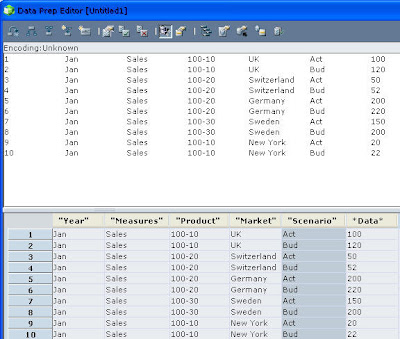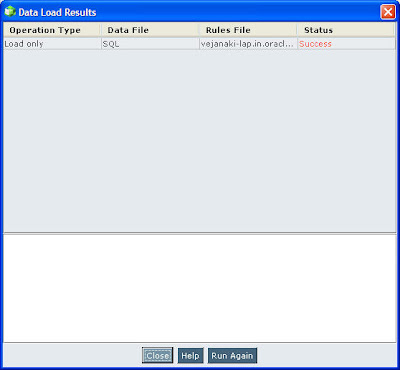Now, let us load the rates (the rates that we saw in the last blog entry) using a similar rules file as above.Once the rate load is done, the next step is to convert the Local currency into the corresponding USD currency. This is achieved through calculation scripts. In effect, the Members of the Scenario dimension under Local, would store the local currency. And the Members of the Scenarion dimension under Dollars, would store the converted USD. To achieve this, let us create a simple calculation script as shown below

SET CACHE HIGH;
SET CALCPARALLEL 2;

FIX("Sales",@LEVMBRS("Market",0))
"Actual" = "Act" / "Actual"->"Rate Product";
"Budget" = "Bud" / "Budget"->"Rate Product";
"Actual @ Bud XChg" = "Act" / "Budget"->"Rate Product";
ENDFIX;

FIX("Actual","Budget","Actual @ Bud XChg","Act","Bud")
AGG("Product");
CALC DIM("Year");
ENDFIX;

FIX("Actual","Budget","Actual @ Bud XChg")
AGG("Market");
ENDFIX;

The above script can actually be split into 3 parts. They are

1. Currency Conversion at the lowest level
2. Aggregation of all the relevant dimension for all the Scenarios except Markets
3. Aggregation of the Markets dimension for USD converted Scenario alone.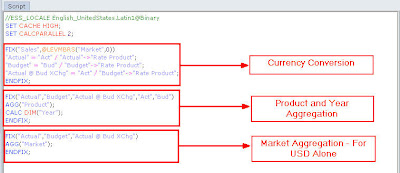The above trifurcation is needed in order to ensure that we are not aggregating Local Currency across Markets as it would not make sense to add Euros with Pounds. Now, let us execute the above calculation and look at the data.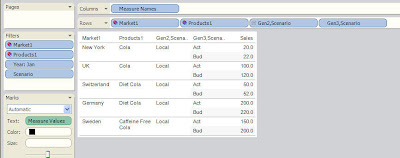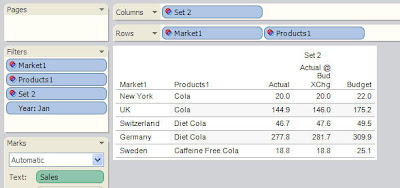As you see, the conversions have happened correctly based on the input rates. The above approach basically overwrites the existing rate values. Hence, after a calculation the rates would not exist in the cube. If rates are desired for future calculations just exclude the “Rate Product” member from the above calculation. The method above can be used/configured to suit quit a few scenarios. But the major drawback with this approach is that there are possibilities of huge amounts of cube fragmentation. Due to the complex nature of the calculations (getting the rates from a specific intersection), unnecessary blocks might be created and hence can result in reduced block density and also cube fragmentation. So, whenever this approach is used, frequent export and import of lowest level data(along with the rollups) is needed.

Posted in All Posts, EPM, Hyperion Essbase | 2 Comments »

Hyperion Essbase 9.3.1 – Alternative Approach to Currency Conversion in BSO Cubes – Using Calculation Scripts – Part 1

Posted by Venkatakrishnan J on December 29, 2008

In our blog series on Essbase, we had seen how to go about building ASO and BSO cubes. We also saw various approaches for loading data, building dimensions using rule files. In the next couple of blog entries we shall see more advanced topics like Currency Coversion in both ASO and BSO cubes. We shall start with the BSO Cube first. BSO cubes support currency conversion using 2 methods

1. Using Currency Database
2. Using inherent Rate Members against each dimension

The first approach is pretty straightforward and is the recommended option while using BSO cubes. This is also documented pretty clearly in the Admin Guide. Unfortunately, ASO does not support a seperate currency database. So, we shall look at an approach today which can be used for both ASO as well as BSO cubes. The actual implementation would vary for ASO and BSO options, but the underlying concept would remain the same. To begin with we shall understand the currency conversion from a BSO outline standpoint. To demonstrate this, we shall be using the Interntl database outline under the Sample Application. The reason for using this specific database is that, it comes with a corresponding currency coversion database which will store the rates. Before we jump into the outline design, we shall understand what exactly are we trying to do. This is summarized in the points below

1. The Interntl database is loaded with data(at local currency for each Market). i.e each record of lowest level data will be coming in Euros, Canadian Dollars, Pounds etc
2. The intention is to convert all the incoming Local Currency data into common reported Dollar which is USD.
3. For this the rates would be loaded against each Market (assuming each Market will have only one currency)
4. The rates would be in the form of a ratio of USD to the Local Currency (For example, USD/GBP etc)

This is just a high level requirement. Our intention is to replicate whatever has been done by the Currency Database using custom inherent Rate members within the database itself. This is achieved using the high level flow listed below

Let us take a look at our base outline first.

As you see, we basically have the below listed dimensions

1. Year
2. Measures (Containing both P/L and B/S accounts)
3. Product
4. Market
5. Scenario

So, our input data should be having the grain of the 5 dimensions above. Since our approach is to load the rates in the same database, we need to identify the grain of the Rates data as well. Our rates depend on the following dimensions

1. Year – Rates would vary over time
2. Measures – Rates would be different for P/L accounts and B/S accounts (in some businesses)
3. Market – Rates would vary based on countries. If the data is stored at city level, rates can be loaded at that level as well. For example, New York would be using USD. Hence while loading the rates one can load the rate directly against New York assuming USD as the base.
4. Scenario – Actual Rates would be different from Budget rates

As you see, in our case Rates have a lesser dimensional grain than the actual data. So, let us list down all the member combinations for each dimension for which rates would be loaded.

1. Year – All lowest level month members (Jan, Feb, Mar, Apr, May. Jun, Jul, Aug, Sep, Oct, Nov, Dec)
2. Measures – All lowest level Account members
3. Product – Rates do not vary based on products. Hence we need to manually add a member called “Rate Product” which will be used for loading rates alone. This is shown in the screenshot below
4. Market – Rates would be loaded against all lowest level members in this dimension.
5. Scenario – Rates would be loaded against Act and Bud members since there are two types of rates that we would be loading.

To give an example of how the rates would be loaded, a set of sample input records is given below

As you see, we are loading the rate against all the possible intersections that we identified above. So, in effect we would be storing the rates in the same database as the actual loaded data. There can be multiple variations of the above approach like using UDA’s for currency to GEO mapping etc but this should give an idea. In the next blog entry we shall see how we can use the above rates to do the data conversion from Local currency to a common USD using Calculation scripts.

Posted in All Posts, EPM, Hyperion Essbase | 3 Comments »